Mathematical and Physical Journal
for High Schools
Issued by the MATFUND Foundation
 Already signed up? New to KöMaL?

# KöMaL Problems in Physics, February 2014

Show/hide problems of signs:## Problems with sign 'M'

Deadline expired on March 10, 2014.

M. 339. Determine what percentage of the volume of a sugar cube is the volume of the sugar.

(6 pont)

statistics## Problems with sign 'P'

Deadline expired on March 10, 2014.

P. 4605. The terminal voltages of the power supplies shown in the figure are constant, and the values of the terminal voltage are: U1=15 V and U2=45 V. The resistances of the resistors are R1=10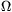and R2=20.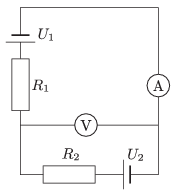a) What are the readings shown by the meters, which are considered ideal?

b) Solve the problem, if the two meters are swapped over.

(3 pont)

solution (in Hungarian), statistics

P. 4606. The motion of a pointlike mass can be split to two parts. In the first part its average speed is v1, and in the second its average speed is v2. For the whole motion the average speed is the geometric mean of the speeds v1 and v2, which is:. Determine the ratio of the covered distances in the two parts of the motion.

(4 pont)

solution (in Hungarian), statistics

P. 4607. The bow of a police boat rises high above the water, whilst the boat is hurrying to a certain place. Does the amount of rise depend on the speed of the boat? Sketch the forces exerted on the boat.

(4 pont)

solution (in Hungarian), statistics

P. 4608. In order that the draught does not slam the door, a flat cylinder shaped object is placed between the door and the threshold, preferably close to the hinge of the door. The symmetry axis is of the cylinder is vertical. To what angle can the draught close the door if the coefficient of kinetic friction between the door and the object is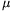1 and that of between the object and the threshold is2?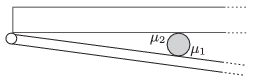(5 pont)

solution (in Hungarian), statistics

P. 4609. Both ends of a horizontal thin tube are closed. There is a mercury thread of length h at the middle of the tube. The length of the air columns in both parts is, and the pressure of both air columns is equal to the gauge pressure of a mercury column of height H. The tube is placed to a tumble dryer, the symmetry axis of which is vertical, and the tumble drier is started to spin.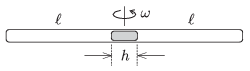Give the displacement of the mercury thread as a function of the angular speed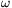, if the temperature is constant.

(5 pont)

solution (in Hungarian), statistics

P. 4610. There is a sample of ideal gas in a cylinder shaped container. The symmetry axis of the cylinder is horizontal and at one end it is closed by frictionlessly moveable piston. Initially the volume of the gas is V1, its temperature is T1 and its pressure is p1, which is the same as the ambient (and later on as well constant) air pressure.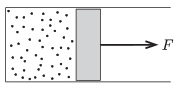The sample of gas is taken through the following processes n times: It is heated at constant pressure to a temperature of T2, and then it is cooled back to the temperature of T1 keeping its volume constant at its reached value. While the gas is heated at constant pressure an external constant force is exerted on the piston, which force is different at the different heating steps. During the whole process the total work done is W.

a) To what value will the volume of the gas increase after the n-th step?

b) What is the total work W done by the external force?

(5 pont)

solution (in Hungarian), statistics

P. 4611. An insulating rubber thread of spring constant D is attached to the ceiling of a room of height h. The floor of the room has a big enough area, and it is made of metal, which is earthed. A light small metal sphere is attached to the other end of the insulating rubber thread; the original length of the rubber thread is. If the sphere is charged to Q then the length of the thread increases to a length of 0.75. Is it possible to increase the charge on the sphere very slowly to 3Q, such that the sphere does not touch the floor? (The electrostatic effect of the ceiling and the walls are negligible.)

(5 pont)

solution (in Hungarian), statistics

P. 4612. An object, which is at a distance of 30 cm from us, is observed through a lens. When the lens is close either to us or to the object then the object seems to have its original size, but when the lens is in between these two positions the object appears bigger.

a) Where is the lens when the object appears the greatest?

b) What is the focal length of the lens, if when the object appears the greatest, its observed size is twice as big as its original size?

(5 pont)

solution (in Hungarian), statistics

P. 4613. A uniform, 1-metre long uninsulated resistance wire, the cross section of which is everywhere constant, is connected to a power supply, the terminal voltage of which is stabilized. One terminal of a resistor, whose resistance is the same as the resistance wire is connected to the end of the resistance wire, whilst the other terminal is moved along the resistance wire.

Where is the pointer of the moving terminal, when the power dissipated on the resistor is one-quarterth of the maximum power dissipated at the resistor?

(4 pont)

solution (in Hungarian), statistics

P. 4614. The linear charge density of a very long, straight, vertical insulating thread is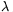=8.10-7 C/m. A very small metal ball of charge q=6.81.10-8 C is suspended by a very thin insulating thread of length=10 cm, which is at a distance of d0=5 cm from the other thread. The metal ball is fixed with an insulating equipment at the position in which the thread is vertical. At a given moment the ball is released from its fixed position without any push. Determine

a) the maximum distance between the vertical metal thread and the ball;

b) the angle between the vertical and the supporting thread of the ball, when the speed of the ball is maximum.

(5 pont)

solution (in Hungarian), statistics

P. 4615. A slinky, a helical spring (used as a toy which is able to travel down a flight of steps) whose unstretched length is negligibly small, obeys Hooke's law with a good approximation, and it has a considerable elongation due to its own weight.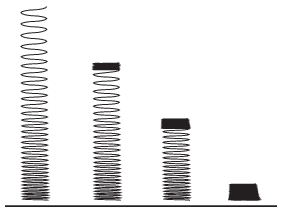a) The slinky of mass m resting on the table is slowly raised at its top end until its lower end just raised from the table. The length of the spring at this position is L. How much work was done during lifting the spring?

b) If the slinky is released from this position, interestingly its lowermost turn does not move until the whole spring reaches its totally compressed length, (see the figure). What is the initial speed of the slinky at which it begins to fall right after reaching its totally compressed position?

(6 pont)

solution (in Hungarian), statistics